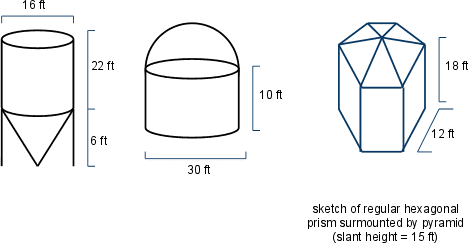# Stokes's Theorem problem

## Homework Statement

http://puu.sh/d5FpW.png [Broken]

## Homework Equations

∫∫(∇XF)⋅dS = ∫F(r(t))⋅r'(t)dt

## The Attempt at a Solution

So I figured I'd have two line integrals (using Stokes's theorem.

Paramatizing S1:

I can think of the cylinder as the closed curve circle x²+y² = 9 on the x-y plane right?

x = 3cost, y = 3sint, z = 0; 0 ≤ t ≤ 2pi

or r(t) = (3cost)i + (3sint)j

Then F = (27(cost)^3)i +(2187(sint)^7)j

The dot product of F and r'(t) gives me

-81sint(cost)3 + 6561(sint)^7cost

Then I integrate this from t = 0 to t = 2pi and get 0.

For S2:

Similar to S1, I can think of this hemisphere as the circle

x²+y² = 9 on the x-y plane with z = 8 right?

Then paramatizing:

r(t) = (3cost)i + (3sint)j + 8k ; 0≤t≤2pi

I go through the same process as S1, but I don't get 0 I get -576 pi, which is obviously wrong.

Would someone mind walking me through how to do this problem correctly?Am I applying Stokes's theorem incorrectly.

Last edited by a moderator:

## Answers and Replies

LCKurtz
Science Advisor
Homework Helper
Gold Member
The only boundary of the silo/hemisphere is the circle in the xy plane, and you got zero for that.

Hi LCKurtz, thanks for your reply.

I was thinking I may only need to do the integral for S1 since, as you said, both surfaces share a circle in the xy plane as the boundary, but thought that made the problem too trivial.

So instead of splitting the surface into two parts S1 and S2, I can think of it as one surface like the middle image in this picture:so what I calculated for S1 is for the entire surface as well?

LCKurtz
Science Advisor
Homework Helper
Gold Member
Yes. One way to think of it is if that surface was flexible rubber or a soap bubble, it could contract down to just the disk inside that circle. Any way you deform the surface still has that boundary.

•izelkay